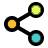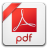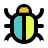Math Calculators, Lessons and Formulas

It is time to solve your math problem

mathportal.org

# Scientific notation calculator

This calculator supports multiplication and division numbers in scientific notation. The calculator supports conversion from scientific notation to decimal, and vice versa. The calculator will generate a detailed step-by-step explanation for each operation.

problem

Divide $5.2\cdot 10^{21}$ by $4\cdot 10^{11}$. Express the result using 10 significant digits.

solution

$$\frac{ 5.2\cdot 10^{21} }{ 4\cdot 10^{11} } = 1.3\cdot 10^{10}$$

explanation

Step 1. Write entered numbers into the scientific notation. (see SN Converter for detailed explanation)

$$5.2\cdot 10^{21} = 5.2\cdot 10^{21}$$$$4\cdot 10^{11} = 4\cdot 10^{11}$$

Step 2. Divide numeric parts:

$$\frac{ 5.2 }{ 4 } = 1.3$$

Step 3. Divide $10^{ 21 }$ by $10^{ 11 }$:

$$\frac{ 10^{ 21 } }{ 10^{ 11 } } = 10^{ 21 - 11 } = 10^{ 10 }$$

Step 4. Write the answer as the product of the numbers obtained in steps 2 and 3:

$$1.3 \cdot 10^{ 10 }$$

## Report an Error !

Script name : scientific-notation-calculator

Form values: 1 , 5.2e21 , 2 , 4e11 , 1 , g ,

Comment (optional)

## Share Result

Or just copy and paste the link wherever you need it.Scientific Notation Converter
show help ↓↓ examples ↓↓
Hide steps
Multiply and divide scientific numbers
show help ↓↓ examples ↓↓
Hide steps
working...
examples
example 1:ex 1:
Write number 73000000 in scientific notation.
example 2:ex 2:
Convert number $8.32 \cdot 10^6$ to decimal notation.
example 3:ex 3:
$$2.5 \cdot 10^{12} \, \cdot \, 3.42 \cdot 10^{-7} =$$
example 4:ex 4:
$$\frac{8.5 \cdot 10^{24}}{2.41 \cdot 10^{18}} =$$
example 5:ex 5:
$$\frac{3 \cdot 10^{-8}}{5.45 \cdot 10^{-6}} =$$
Search our database of more than 200 calculators